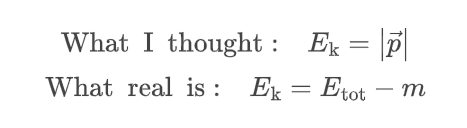# Kinetic energy in Compton process

In the Compton process (e.g. `G4KleinNishinaCompton`), the final kinetic energy of the electron is evaluted by E_k,electron = E_gamma,initial - E_gamma,final.

However, from the relativistic dynamics’ point of view, it is the total energy not the kinetic energy that conserved during interaction. Thus we should have E_total,electron = E_gamma,initial - E_gamma,final + m_electron. And then we can evaluate E_k,electron = sqrt(E_total,electron^2 - m_electron^2).

Do I miss some thing here or is Geant4 not accuracte at low energy scale (~1MeV)?

Ciao,

I am not sure that understand the point here: in the Compton scattering, the e- already exists (and it is an atomic electron), so this contribution is the same for the initial and the final state. So, in this case, the total kinetic energy is conserved, in addition to the total relativistic energy.

Ciao,
Luciano

1 Like

Ciao Luciano,

In the relativistic scenario, we still conservation of the summed momentum. It has the following vector form:

But this is not the same as the G4’s implementation.

Best,
Fan

" And then we can evaluate E_k,electron = sqrt(E_total,electron^2 - m_electron^2) "

I believe the correct equation must be: p = sqrt (Etotˆ2 - mˆ2)

As Luciano wrote, the first equation is just total energy conservation :
(Egamma + Etot e-) initial = (Egamma + Etot e-) final

Etot e- initial = m —> electron target at rest
Etot e- final = m + Ekin e-

1 Like

Thanks for pointing out the reference, it really helps.

I finally found that it is my understanding to kinetic energy in relativistic dynamics is wrong:You and pandola are right, relativistic kinetic energy is conserved.

@Adiolph good that the issue was sorted it out. Thanks for the discussion,

This topic was automatically closed 7 days after the last reply. New replies are no longer allowed.